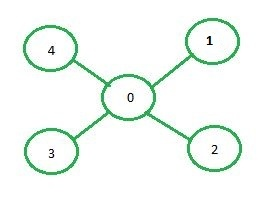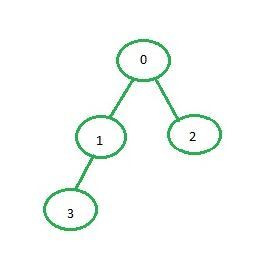Related Articles

# Implementation of DFS using adjacency matrix

• Difficulty Level : Medium
• Last Updated : 19 Aug, 2020

Adjacency matrix representation: In adjacency matrix representation of a graph, the matrix mat[][] of size n*n (where n is the number of vertices) will represent the edges of the graph where mat[i][j] = 1 represents that there is an edge between the vertices i and j while mat[i][i] = 0 represents that there is no edge between the vertices i and j.Below is the adjacency matrix representation of the graph shown in the above image:

```   0 1 2 3 4
0  0 1 1 1 1
1  1 0 0 0 0
2  1 0 0 0 0
3  1 0 0 0 0
4  1 0 0 0 0
```

Examples:

```Input: source = 0Output: 0 1 3 2

Input: source = 0Output: 0 1 2 3 4
```

## Recommended: Please try your approach on {IDE} first, before moving on to the solution.

Approach:

• Create a matrix of size n*n where every element is 0 representing there is no edge in the graph.
• Now, for every edge of the graph between the vertices i and j set mat[i][j] = 1.
• After the adjacency matrix has been created and filled, call the recursive function for the source i.e. vertex 0 that will recursively call the same function for all the vertices adjacent to it.
• Also, keep an array to keep track of the visited vertices i.e. visited[i] = true represents that vertex i has been been visited before and the DFS function for some already visited node need not be called.

Below is the implementation of the above approach:

## C++

 `// C++ implementation of the approach``#include ``using` `namespace` `std;`` ` `class` `Graph {`` ` `    ``// Number of vertex``    ``int` `v;`` ` `    ``// Number of edges``    ``int` `e;`` ` `    ``// Adjacency matrix``    ``int``** adj;`` ` `public``:``    ``// To create the initial adjacency matrix``    ``Graph(``int` `v, ``int` `e);`` ` `    ``// Function to insert a new edge``    ``void` `addEdge(``int` `start, ``int` `e);`` ` `    ``// Function to display the DFS traversal``    ``void` `DFS(``int` `start, vector<``bool``>& visited);``};`` ` `// Function to fill the empty adjacency matrix``Graph::Graph(``int` `v, ``int` `e)``{``    ``this``->v = v;``    ``this``->e = e;``    ``adj = ``new` `int``*[v];``    ``for` `(``int` `row = 0; row < v; row++) {``        ``adj[row] = ``new` `int``[v];``        ``for` `(``int` `column = 0; column < v; column++) {``            ``adj[row][column] = 0;``        ``}``    ``}``}`` ` `// Function to add an edge to the graph``void` `Graph::addEdge(``int` `start, ``int` `e)``{`` ` `    ``// Considering a bidirectional edge``    ``adj[start][e] = 1;``    ``adj[e][start] = 1;``}`` ` `// Function to perform DFS on the graph``void` `Graph::DFS(``int` `start, vector<``bool``>& visited)``{`` ` `    ``// Print the current node``    ``cout << start << ``" "``;`` ` `    ``// Set current node as visited``    ``visited[start] = ``true``;`` ` `    ``// For every node of the graph``    ``for` `(``int` `i = 0; i < v; i++) {`` ` `        ``// If some node is adjacent to the current node``        ``// and it has not already been visited``        ``if` `(adj[start][i] == 1 && (!visited[i])) {``            ``DFS(i, visited);``        ``}``    ``}``}`` ` `// Driver code``int` `main()``{``    ``int` `v = 5, e = 4;`` ` `    ``// Create the graph``    ``Graph G(v, e);``    ``G.addEdge(0, 1);``    ``G.addEdge(0, 2);``    ``G.addEdge(0, 3);``    ``G.addEdge(0, 4);`` ` `    ``// Visited vector to so that``    ``// a vertex is not visited more than once``    ``// Initializing the vector to false as no``    ``// vertex is visited at the beginning``    ``vector<``bool``> visited(v, ``false``);`` ` `    ``// Perform DFS``    ``G.DFS(0, visited);``}`

## Python3

 `# Python3 implementation of the approach ``class` `Graph:``     ` `    ``adj ``=` `[]`` ` `    ``# Function to fill empty adjacency matrix``    ``def` `__init__(``self``, v, e):``         ` `        ``self``.v ``=` `v``        ``self``.e ``=` `e``        ``Graph.adj ``=` `[[``0` `for` `i ``in` `range``(v)] ``                        ``for` `j ``in` `range``(v)]`` ` `    ``# Function to add an edge to the graph``    ``def` `addEdge(``self``, start, e):``         ` `        ``# Considering a bidirectional edge``        ``Graph.adj[start][e] ``=` `1``        ``Graph.adj[e][start] ``=` `1`` ` `    ``# Function to perform DFS on the graph``    ``def` `DFS(``self``, start, visited):``         ` `        ``# Print current node``        ``print``(start, end ``=` `' '``)`` ` `        ``# Set current node as visited``        ``visited[start] ``=` `True`` ` `        ``# For every node of the graph``        ``for` `i ``in` `range``(``self``.v):``             ` `            ``# If some node is adjacent to the ``            ``# current node and it has not ``            ``# already been visited``            ``if` `(Graph.adj[start][i] ``=``=` `1` `and``                    ``(``not` `visited[i])):``                ``self``.DFS(i, visited)`` ` `# Driver code``v, e ``=` `5``, ``4`` ` `# Create the graph``G ``=` `Graph(v, e)``G.addEdge(``0``, ``1``)``G.addEdge(``0``, ``2``)``G.addEdge(``0``, ``3``)``G.addEdge(``0``, ``4``)`` ` `# Visited vector to so that a vertex``# is not visited more than once``# Initializing the vector to false as no``# vertex is visited at the beginning``visited ``=` `[``False``] ``*` `v`` ` `# Perform DFS``G.DFS(``0``, visited);`` ` `# This code is contributed by ng24_7`
Output:
```0 1 2 3 4
```

Attention reader! Don’t stop learning now. Get hold of all the important DSA concepts with the DSA Self Paced Course at a student-friendly price and become industry ready.  To complete your preparation from learning a language to DS Algo and many more,  please refer Complete Interview Preparation Course.

In case you wish to attend live classes with experts, please refer DSA Live Classes for Working Professionals and Competitive Programming Live for Students.

My Personal Notes arrow_drop_up